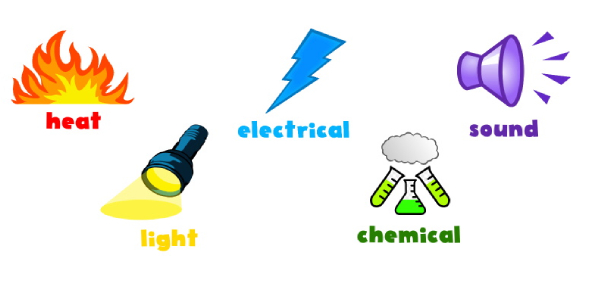# Forms Of Energy: Trivia Exam!

10 Questions | Attempts: 264
ShareSettingsEver wondered how much you know about the various forms of energy? The different forms of energy consist of mechanical energy, thermal energy, nuclear energy, chemical energy, and electromagnetic energy. As far as this quiz is concerned, the definition of energy determines that it can do what, which form of energy involves the flow of electrons toward protons, what are atomic vibrations, and which form of energy consists of the movement of objects. Just remember to secure your certificate when you are done with the quiz.

• 1.
The definition of energy determines that it can do what?
• A.

Destroy things

• B.

Cause changes

• C.

Do work

• D.

Create things

• 2.
Which form of energy involves the flow of electrons toward protons?
• A.

Thermal

• B.

Nuclear

• C.

Chemical

• D.

Electromagnetic

• E.

Mechanical

• 3.
Which form of energy involves the atomic vibrations?
• A.

Thermal

• B.

Chemical

• C.

Electromagnetic

• D.

Nuclear

• E.

Mechanical

• 4.
Which form of energy involves the movement of objects?
• A.

Thermal

• B.

Chemical

• C.

Electromagnetic

• D.

Nuclear

• E.

Mechanical

• 5.
Which form of energy involves breaking down or forming chemical bonds?
• A.

Thermal

• B.

Chemical

• C.

Electromagnetic

• D.

Nuclear

• E.

Mechanical

• 6.
Which form of energy involves the fusing or splitting of atomic nuclei?
• A.

Thermal

• B.

Chemical

• C.

Electromagnetic

• D.

Nuclear

• E.

Mechanical

• 7.
Dissolving sugar in water is a chemical change.
• A.

True

• B.

False

• 8.
Select all options that are CHEMICAL changes.
• A.

Melting

• B.

Digesting

• C.

Burning

• D.

Eroding

• E.

Rusting

• F.

Decaying

• G.

Ripening (fruit)

• 9.
Light and solar rays are both forms of electromagnetic energy.
• A.

True

• B.

False

## Related TopicsBack to top
×

Wait!
Here's an interesting quiz for you.